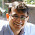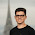## Saturday, January 16, 2016

### How to Optimize Json.NET Serialization PerformanceNewtonsoft is a pretty fast JSON serializer, but you can make it even faster!

By default, JsonConvert uses reflection to recursively search through the structure of an object during the serialization process. By implementing a custom JsonConverter that already knows the exact structure of the object, you can significantly increase serialization performance.

How much faster? That depends! The more complicated the data structure, the larger the performance gain. Below is a simple example...

Action Method Milliseconds Performance Increase
Serialize Standard 1134 115.59%
Custom 526
Deserialize Standard 1488 62.98%
Custom 913

### Model and Converter

`public class Model`
`{`
`    public int Int { get; set; }`
` `
`    public bool Bool { get; set; }`
` `
`    public string String { get; set; }`
`}`
` `
`public class ModelConverter : JsonConverter`
`{`
`    public static readonly ModelConverter Instance = new ModelConverter();`
` `
`    private static readonly Type ModelType = typeof(ICollection<Model>);`
` `
`    public override bool CanConvert(Type objectType)`
`    {`
`        return ModelType.IsAssignableFrom(objectType);`
`    }`
` `
`    public override void WriteJson(`
`        JsonWriter writer,`
`        object value,`
`        JsonSerializer serializer)`
`    {`
`        var collection = (ICollection<Model>)value;`
` `
`        writer.WriteStartArray();`
` `
`        foreach (var model in collection)`
`        {`
`            writer.WriteStartObject();`
` `
`            writer.WritePropertyName("Int");`
`            writer.WriteValue(model.Int);`
` `
`            writer.WritePropertyName("Bool");`
`            writer.WriteValue(model.Bool);`
` `
`            writer.WritePropertyName("String");`
`            writer.WriteValue(model.String);`
` `
`            writer.WriteEndObject();`
`        }`
` `
`        writer.WriteEndArray();`
`    }`
` `
`    public override object ReadJson(`
`        JsonReader reader,`
`        Type objectType,`
`        object existingValue,`
`        JsonSerializer serializer)`
`    {`
`        var collection = new List<Model>();`
` `
`        Model model = null;`
` `
`        while (reader.Read())`
`        {`
`            switch (reader.TokenType)`
`            {`
`                case JsonToken.StartObject:`
`                    model = new Model();`
`                    collection.Add(model);`
`                    break;`
` `
`                case JsonToken.PropertyName:`
`                    SetProperty(reader, model);`
`                    break;`
` `
`                case JsonToken.EndArray:`
`                    return collection;`
`            }`
`        }`
` `
`        return collection;`
`    }`
` `
`    private static void SetProperty(JsonReader reader, Model model)`
`    {`
`        var name = (string)reader.Value;`
` `
`        reader.Read();`
` `
`        switch (name)`
`        {`
`            case "Int":`
`                model.Int = Convert.ToInt32(reader.Value);`
`                break;`
` `
`            case "Bool":`
`                model.Bool = (bool)reader.Value;`
`                break;`
` `
`            case "String":`
`                model.String = (string)reader.Value;`
`                break;`
`        }`
`    }`
`}`

### Unit Tests

`public class JsonConverterPerformanceTests`
`{`
`    private readonly ITestOutputHelper _output;`
` `
`    public JsonConverterPerformanceTests(ITestOutputHelper output)`
`    {`
`        _output = output;`
`    }`
` `
`    [Fact]`
`    public void ModelConverterPerformance()`
`    {`
`        var collection = Enumerable`
`            .Range(1, 100)`
`            .Select(i => new Model`
`            {`
`                Int = i,`
`                Bool = i % 2 == 0,`
`                String = "Hello World"`
`            })`
`            .ToList();`
` `
`        const int iterations = 10000;`
` `
`        var json = JsonConvert.SerializeObject(collection, ModelConverter.Instance);`
` `
`        var sw1 = Stopwatch.StartNew();`
`        for (var i = 0; i < iterations; i++)`
`            JsonConvert.SerializeObject(collection);`
`        sw1.Stop();`
` `
`        _output.WriteLine("Standard Serialize: " + sw1.ElapsedMilliseconds);`
` `
`        var sw2 = Stopwatch.StartNew();`
`        for (var i = 0; i < iterations; i++)`
`            JsonConvert.SerializeObject(collection, ModelConverter.Instance);`
`        sw2.Stop();`
` `
`        _output.WriteLine("Custom Serialize: " + sw2.ElapsedMilliseconds);`
` `
`        var sw3 = Stopwatch.StartNew();`
`        for (var i = 0; i < iterations; i++)`
`            JsonConvert.DeserializeObject<Model[]>(json);`
`        sw3.Stop();`
` `
`        _output.WriteLine("Standard Deserialize: " + sw3.ElapsedMilliseconds);`
` `
`        var sw4 = Stopwatch.StartNew();`
`        for (var i = 0; i < iterations; i++)`
`            JsonConvert.DeserializeObject<ICollection<Model>>(json, ModelConverter.Instance);`
`        sw4.Stop();`
` `
`        _output.WriteLine("Custom Deserialize: " + sw4.ElapsedMilliseconds);`
` `
`        Assert.True(sw1.ElapsedMilliseconds > sw2.ElapsedMilliseconds);`
`        Assert.True(sw3.ElapsedMilliseconds > sw4.ElapsedMilliseconds);`
`    }`
`}`

Enjoy,
Tom

1.take a look at this - https://github.com/kevin-montrose/Jil

1.This is very interesting, thank you for sharing!

Keep in mind that the performance metrics provided in that repository are using the default Newtonsoft serializers. This means that with the optimizations from this blog post they would be far more comparable, possibly even in favor of Newtonsoft.

2.This example is very dependent of the model. If you have several models, it doesn't seem to be a best practice, to build a Converter for each Model. To make this conversion more generic by receiving generic objects, probably using Reflection, Would it be possible to achieve the same optimal performance?

1.You are correct that this implementation is very dependent on the model, which is the whole point and where the performance gain comes from. I could imaging using code generation to optimize the process of creating converters, but I am not sure you could get as good performance otherwise.

3.But does JSON.Net cache the info in anyway so next time it doesn't use reflection?

1.Yes, the DefaultContractResolver does cache the contract look ups: https://github.com/JamesNK/Newtonsoft.Json/blob/master/Src/Newtonsoft.Json/Serialization/DefaultContractResolver.cs#L235

Regardless of the cache, Newtonsoft still needs to process those results, and that takes time. By having a hard coded converter the application can just directly process serialization and avoid any overhead (no matter how small) of trying to dynamically serialize properties and values. This is a classic performance problem of dynamic versus static, and static is always going to be faster.

Please do not misunderstand me, Newtonsoft is absolutely amazing and should be applauded for both it's design and performance! This is just an optimization for extreme performance needs.

4.I wonder how would you do if there was a really huge array in that model and how would you implement so that the array serialization had to be deferred into a IEnumerable.

Nice! If there were a lib that generates code just like XmlSerilizer does for each new type being serialized/deserialized, it would be awesome!